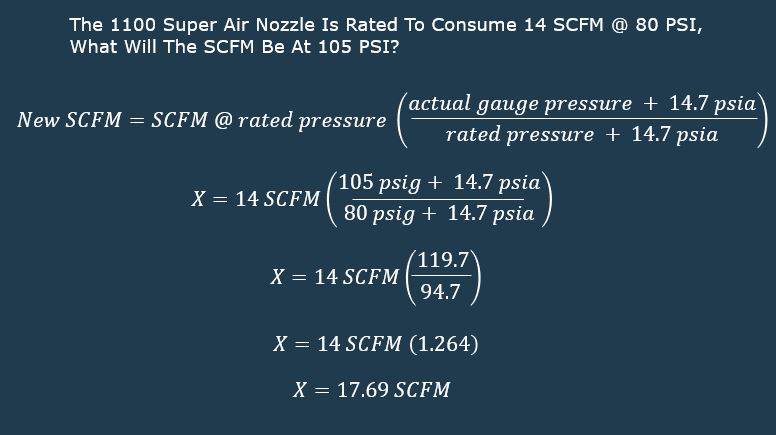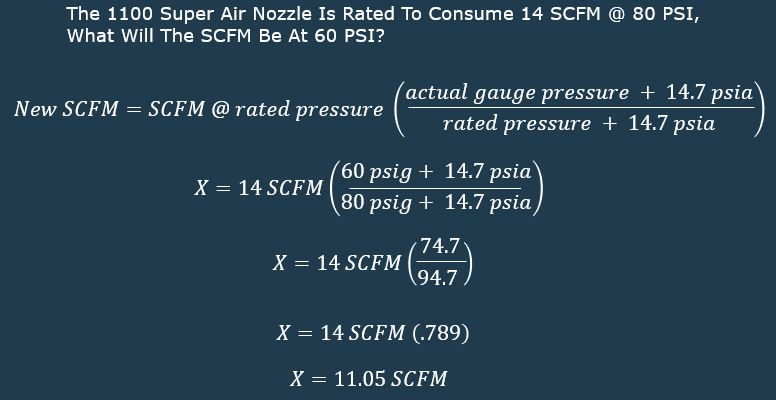## Video Blog: How To Calculate Air Consumption At A Pressure Other Than Published Values

The below video shows how to calculate the air consumption when operating at any pressure.

If you want to discuss efficient compressed air use or any of EXAIR’s engineered compressed air products, give us a call or email.  We would enjoy hearing from you!

Steve Harrison
Application Engineer
Send me an email
Find us on the Web

## How to Calculate SCFM (Volume) When Operating at Any Pressure

If you need to operate at a different pressure because you require less or more force or simply operate at a different line pressure, this formula will allow you to determine the volume of air being consumed by any device.Using the EXAIR 1100 Super Air Nozzle as our example:Lets first consider the volume of the 1100 Super Air Nozzle at a higher than published pressure.  As shown in the formula and calculations it is simply the ratio of gauge pressure + atmospheric divided by the published pressure + atmospheric and then multiply the dividend by the published volume.  So as we do the math we solve for 17.69 SCFM @ 105 PSIG from a device that was  shown consume 14 SCFM @ 80 PSIG.Now lets consider the volume at a lower than published pressure.  As shown it is simply the ratio of gauge pressure + atmospheric divided by the published pressure + atmospheric and then multiply the dividend by the published volume.  So as we do the math we solve for 11.04 SCFM @ 60 PSIG from a device that was shown to consume 14 SCFM @ 80 PSIG.When you are looking for expert advice on safe, quiet and efficient point of use compressed air products give us a call.  Experience the EXAIR difference first hand and receive the great customer service, products and attention you deserve!  We would enjoy hearing from you.

Application Engineer
Send me an email
Find us on the Web